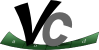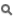Vc  1.1.0 SIMD Vector Classes for C++simdize<T>

Detailed Description

Automatic type vectorization.

The simdize<T> expression transforms the type T to a vectorized variant. This requires the type T to be a class template instance.

Example: First, we declare a class template for a three-dimensional point. The template parameter T determines the type of the members and is float in the scalar (classical) case.

template <typename T> class PointTemplate
{
T x, y, z;
using Instance = PointTemplate<T>;
Vc_SIMDIZE_MEMBER(T, 0, x); // Makes the members accessible via get<N>(point), allowing the
Vc_SIMDIZE_MEMBER(T, 1, y); // simdize implementation to convert between Point and PointV (see
Vc_SIMDIZE_MEMBER(T, 2, z); // below).
public:
Vc_SIMDIZE_STRUCT(Instance, 3); // This declares the actual non-member get and tuple_size.
PointTemplate(T xx, T yy, T zz) : x{xx}, y{yy}, z{zz} {};
// The following function is will automatically be vectorized in the PointV type.
T distance_to_origin() const {
return std::sqrt(x * x + y * y + z * z);
}
};

In the following we create a type alias for the scalar type, which simply means instantiating PointTemplate with float. The resulting type can then be transformed with simdize.

using Point = PointTemplate<float>; // A simple struct with three floats and two functions.
using PointV = Vc::simdize<Point>; // The vectorization of Point stores three float_v and thus
// float_v::size() Points.

The following shows a code example using the above Point and PointV types.

PointV pv = Point{0.f, 1.f, 2.f}; // Constructs a PointV containing PointV::size()
// copies of Point{0, 1, 2}.
for (int i = 1; i < int(pv.size()); ++i) {
assign(pv, i, {i + 0.f, i + 1.f, i + 2.f});
}
const Vc::float_v l = pv.distance_to_origin();
std::cout << l << '\n';
// prints [2.23607, 3.74166, 5.38516, 7.07107, 8.77496, 10.4881, 12.2066, 13.9284] with
// float_v::size() == 8
const Point most_distant = extract(pv, (l.max() == l).firstOne());
std::cout << '(' << most_distant.x << ", " << most_distant.y << ", " << most_distant.z << ")\n";
// prints (7, 8, 9) with float_v::size() == 8

Classes

class  Iterator< T, N, M, V, Size, std::bidirectional_iterator_tag >
This is the iterator type created when applying simdize to a bidirectional iterator type. More...

class  Iterator< T, N, M, V, Size, std::random_access_iterator_tag >
This is the iterator type created when applying simdize to a random access iterator type. More...

Macros

#define Vc_SIMDIZE_INTERFACE(MEMBERS_)

Typedefs

template<typename T , size_t N = 0, typename MT = void>
using simdize = SimdizeDetail::simdize< T, N, MT >

Functions

template<typename S , typename T , size_t N>
void assign (Adapter< S, T, N > &a, size_t i, const S &x)
Assigns one scalar object x to a SIMD slot at offset i in the simdized object a.

template<typename S , typename T , size_t N>
extract (const Adapter< S, T, N > &a, size_t i)
Extracts and returns one scalar object from a SIMD slot at offset i in the simdized object a.

template<typename S , typename T , size_t N>
Adapter< S, T, N > shifted (const Adapter< S, T, N > &a, int shift)
Returns a new vectorized object where each entry is shifted by shift. More...

template<typename S , typename T , std::size_t N>
void swap (Adapter< S, T, N > &a, std::size_t i, S &x)
Swaps one scalar object x with a SIMD slot at offset i in the simdized object a.

template<typename A >
void swap (Scalar< A > &&a, typename A::scalar_type &b)
std::swap interface to swapping one scalar object with a (virtual) reference to another object inside a vectorized object

template<typename A >
void swap (typename A::scalar_type &b, Scalar< A > &&a)
std::swap interface to swapping one scalar object with a (virtual) reference to another object inside a vectorized object

Macro Definition Documentation

 #define Vc_SIMDIZE_INTERFACE ( MEMBERS_ )
Value:
template <std::size_t N_> \
inline auto vc_get_()->decltype(std::get<N_>(std::tie MEMBERS_)) \
{ \
return std::get<N_>(std::tie MEMBERS_); \
} \
template <std::size_t N_> \
inline auto vc_get_() const->decltype(std::get<N_>(std::tie MEMBERS_)) \
{ \
return std::get<N_>(std::tie MEMBERS_); \
} \
enum : std::size_t { \
tuple_size = std::tuple_size<decltype(std::tie MEMBERS_)>::value \
}
Definition: vector.h:258

Declares functions and constants for introspection by the simdize functions. This allows e.g. conversion between scalar T and simdize<T>.

Parameters
 MEMBERS_ The data members of this struct/class listed inside extra parenthesis. The extra parenthesis are required because the macro would otherwise see a variable number of arguments.

Example:

template <typename T, typename U> struct X {
T a;
U b;
};
Note
You must use this macros in the public section of a class.

Definition at line 1693 of file simdize.h.

Typedef Documentation

 using simdize = SimdizeDetail::simdize

Vectorize/Simdize the given type T.

Template Parameters
 T This type must be a class template instance where the template arguments can be recursively replaced with their vectorized variant. If the type implements a specific interface for introspection and member modification, the resulting type can easily be constructed from objects of type T and scalar objects of type T can be extracted from it. N This value determines the width of the vectorization. Per default it is set to 0 making the implementation choose the value considering the compilation target and the given type T. MT This type determines the type to be used when replacing bool with Mask. If it is set to void the implementation choosed the type as smart as possible.
Vc_SIMDIZE_STRUCT, Vc_SIMDIZE_MEMBER

Definition at line 1672 of file simdize.h.

Function Documentation

 Adapter Vc::SimdizeDetail::shifted ( const Adapter< S, T, N > & a, int shift )
inline

Returns a new vectorized object where each entry is shifted by shift.

This basically calls Vector<T>::shifted on every entry.

Parameters
 a The object to apply the shift on. shift The number of entries to shift by.
Returns
a copy of a shifted by shift.

Definition at line 999 of file simdize.h.